Worksheets and No Prep Teaching Resources
Math Worksheets

# Odd and Even Numbers Worksheets

The concepts of odd and even numbers might seem odd to students, but not if they have these clear, all-inclusive worksheets to help them. Visually stunning worksheets will thrill younger students and give them a clear picture of odd and even numbers in a fun way!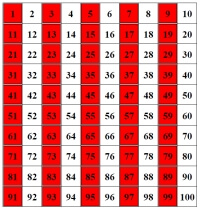Counting and Odd or Even Numbers: Numbers 0 to 9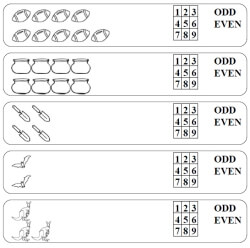Counting and Circle the Number; Circle Odd or Even (images in 2 rows) Counting and Circle the Number; Circle Odd or Even Counting and Write the Number; Write Odd or Even (first problem has choices to circle)

Odd and Even Math Activity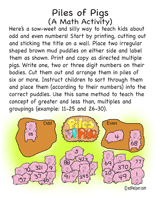Piles of Pigs

Odd or Even Activity Book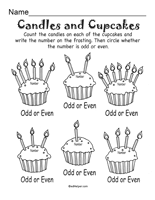Odd or Even Activity Book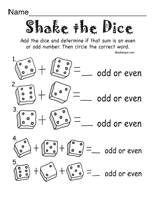Odd or Even Activity Book #2

Counting and Odd or Even Numbers: Numbers 10 to 21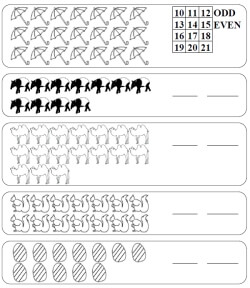Counting and Circle the Number; Circle Odd or Even Counting and Write the Number; Write Odd or Even (first problem has choices to circle)

Odd Numbers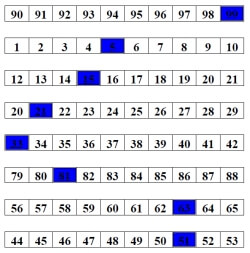Fill-in all of the odd numbers up to 100 Color in the odd numbers up to 100 (5 are given) Color in the odd numbers up to 100 (2 are given) Rows - Color in the odd numbers (1 given in each row) Rows - Color in the odd numbers

Even Numbers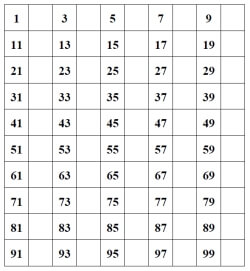Fill-in all of the even numbers up to 100 Color in the even numbers up to 100 (5 are given) Color in the even numbers up to 100 (2 are given) Rows - Color in the even numbers (1 given in each row) Rows - Color in the even numbers

Critical Thinking with Even and Odd Numbers
Number of books (Odd and Even)
Tickets sold by the school drama team
Gold, silver, and bronze medals awarded

Number Patterns
Even and Odd Numbers
Circle odd or even (numbers 1 to 29)
Circle odd or even (numbers 1 to 99)
Write odd or even

Before, After, Between
Just before and after, Just before, Just after
Even and odd numbers: numbers 3 to 29 (easier)
Even and odd numbers: numbers 3 to 29
Even and odd numbers: numbers 10 to 99 (easier)
Even and odd numbers: numbers 10 to 99

Counting
Counting forward and backward (with a number line)
Counting forward and backward

Skip-counting (includes hint on how to skip count)
Skip count by 2's (numbers 2 to 49)

Odd and Even Activity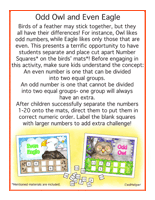Odd Owl and Even Eagle

Odd and Even Activity Book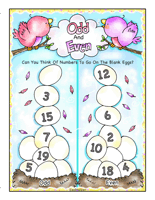Odd And Even

Probability (with the probability of even and odd events)
Theoretical probability (write answer as a decimal)
Theoretical probability (write answer as a percent)

Have a suggestion or would like to leave feedback?